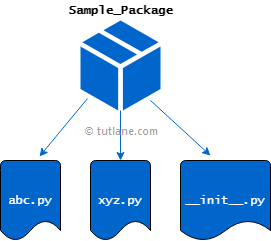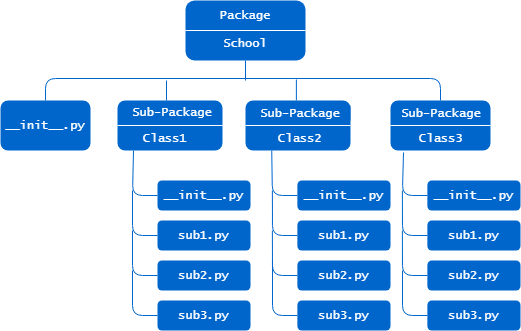# Python Packages

As we learned in the previous characters, modules are the files that will contain the python statements, classes, functions, etc. A package is a combination of one or more module files in a single directory.

Using packages, you can manage a large number of python files in different folders and subfolders based on your requirements.

In python, if you create a directory with python files and an empty `__init__.py` file will be treated as a package. To consider any directory as a package, that directory must contain the `__init__.py` file. By default, the file `__init__.py` is empty, but you can write your package initialization code based on your requirements.

Following is the pictorial representation of creating the package in python.## Create Package in Python

To create a package, first you need to create the directory, and the directory name will be the name of the package. Here, our package name is “Sample_Package” and add your module files inside the directory along with the `__init__.py` file to consider it as a package in python.

The `__init__.py` file can be empty, or it can contain some valid python code. The code whatever you write in the `__init__.py` file will execute whenever the package is imported. So, it's better to write your package initialization code, e.g., import other modules or set some values. Here, we added `abc.py` and `xyz.py` modules files inside the directory with the following code.

### abc.py

return a + b

def subtract(a, b):
return a - b

### xyz.py

def multiply(a, b):
return a * b

def division(a, b):
return a/b

## Import Modules from Package

In python, to access the modules from packages, you need to import the modules along with packages.

Following is the sample way of accessing the modules from packages in python.

from Sample_Package import abc, xyz
or
import Sample_Package.abc
import Sample_Package.xyz

Create the pythonpackages.py file in the same package's directory location and write the following code to access the modules from python packages.

from Sample_Package import abc, xyz

print("Subtract:", abc.subtract(20, 10))
print("Multiply:", xyz.multiply(10, 20))
print("Division:", xyz.division(100, 20))

If you observe the above packages example, we imported both modules (abc, xyz) from the package (Sample_Package) and access the functions from each module.

When you execute the above python packages example, it will return the result as shown below.

Subtract: 10
Multiply: 200
Division: 5.0

Following is another way to access the modules from packages in python.

import Sample_Package.abc
import Sample_Package.xyz

print("Subtract:", Sample_Package.abc.subtract(20, 10))
print("Multiply:", Sample_Package.xyz.multiply(10, 20))
print("Division:", Sample_Package.xyz.division(100, 20))

## Import Specific Functions from Package

If you want, you can import only the specific functions from packages in python as shown below.

from Sample_Package.xyz import multiply

print("Multiply:", multiply(10, 20))

The above python packages example will return the result as shown below.

Multiply: 200

As discussed, every package will contain an empty `__init__.py` file. If required, you can write your package initialization code in the `__init__.py` file to execute while importing the package.

We will write a code in the `__init__.py` file to import the functions directly from the packages instead of modules. Open your `__init__.py` file and write the code as shown below.

### __init__.py

from .xyz import multiply

To test, create the testpkg.py file in the same directory where package (Sample_Package) is located and write the code as shown below.

print("Multiply:", multiply(10, 20))

If you observe the above code, we imported the functions directly from the package instead of modules.

The above python packages example will return the result as shown below.

Multiply: 200

## Create Sub-packages Inside Package

If required, you can also create the sub-packages inside the python package as following based on your requirements.The sub-package module functions can be accessed as shown below.

from School.Class1.sub3 import marks
or
import Sample_Package.Class2.sub2

This is how you can use the python packages to maintain a large number of python files in different folders and subfolders based on your requirements.